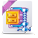# Decision Optimization

View Only

## Solve division of decision variable in objective function by CPLEX#### Farahanim MisniFri June 19, 2020 04:14 AM#### Daniel JunglasFri June 19, 2020 05:09 AM#### Gonzalo Arturo Garcia MorenoWed May 25, 2022 03:55 PM#### Paul RubinWed May 25, 2022 05:38 PM• #### 1.  Solve division of decision variable in objective function by CPLEX

Posted Fri June 19, 2020 04:14 AM
Hi,
I need a help. I need to solve my problem by using CPLEX. My objective function is nonlinear function where in some formulation, there have a division of decision variable. I know CPLEX can not solve nonlinear programming, so I used 'using Cp;' in my file.mod to solve it. It can be solved but unfortunately, the solution given is not an optimal solution. There are some errors in the solution given. Can anybody help me how to solve this problem? Can be any other ways to solve this in order to get exact solution? Here I attach my coding for file.mod. Thank you.

using CP;
int scale=1000;
{string} depot=...;
{string} customer=...;
{string} vehicle=...;
{string} node=...;
int N=card(customer);
int pr=...;
float dist[node][node]=...;
float fcost[depot]=...;
float D[customer]=...;
float R[customer]=...;
float VC[vehicle]=...;
float VD[depot]=...;
dvar boolean x[node][node][vehicle];
dvar boolean z[depot];
dvar boolean y[depot][customer];
//dvar float+ u[customer][vehicle];
dvar int u[customer][vehicle];
dvar int scaleQ[i in depot] in 0..240000;
dexpr float Q[i in depot]=scaleQ[i]/scale;

dexpr float facility=sum(i in node, j in node:j!=i, k in vehicle)x[i][j][k]*dist[i][j];
dexpr float travel=sum(i in depot)fcost[i]*z[i];
dexpr float setup=17*sum(i in depot, j in customer)(D[j]-R[j])*y[i][j]/Q[i];
dexpr float holdinginventory=(1/pr)*sum(i in depot)(Q[i]*pr - Q[i]*sum(j in customer)(D[j]+R[j])*y[i][j]);
dexpr float cost=travel + facility + setup + holdinginventory;

minimize cost;
subject to {
forall(j in customer) sum(i in node, k in vehicle)x[i][j][k]==1;
forall(k in vehicle) sum(i in depot, j in customer)x[i][j][k]<=1;
forall(k in vehicle) sum(i in node, j in customer:j!=i)D[j]*x[i][j][k]<=VC[k];
forall(i in node, k in vehicle) (sum(j in node)x[i][j][k])- (sum(j in node)x[j][i][k])==0;
forall(i in depot) sum(j in customer)D[j]*y[i][j]<=VD[i]*z[i];
forall(j in customer) sum(i in depot)y[i][j]==1;
forall(l in customer, j in customer, k in vehicle) u[l][k]-u[j][k]+N*x[l][j][k]<= N-1;
forall(i in depot, j in customer, k in vehicle) (sum(h in node)(x[i][h][k]+x[h][j][k]))-y[i][j]<=1;
}

------------------------------
Farahanim Misni
------------------------------

• #### 2.  RE: Solve division of decision variable in objective function by CPLEX

Posted Fri June 19, 2020 05:09 AM
Why is the solution produced by CP not optimal? Does CP stop the search before proving optimality?

Division by decision variables is indeed not supported by the CPLEX engine. As far as I can tell, this division is only used in dexpr setup. Can you maybe replace this by a piecewise linear function? That should not be too hard, I think, since Q[i] is not really a float variable. It is integer variable scaleQ[i] divided by 1000. So you immediately get the breakpoints for the pwl function: the points in the domain of scaleQ[i] divided by 1000.

------------------------------
Daniel Junglas
------------------------------

• #### 3.  RE: Solve division of decision variable in objective function by CPLEX

Posted Wed May 25, 2022 03:55 PM
Hi,

I am working in a model where I have 2 non-linear constraints.

The construction of the non-linear constraints is the following:
integer/boolean=float        (decision variables)

How can I solve such a problem?
Thanks!------------------------------
Gonzalo Arturo Garcia Moreno
------------------------------

Attachment(s)ramp-up_question.zip   563 KB 1 version

• #### 4.  RE: Solve division of decision variable in objective function by CPLEX

Posted Wed May 25, 2022 05:38 PM
Assuming your denominator cannot be zero, integer / boolean = float is equivalent to integer = boolean * float. If you go to Operations Research Stack Exchange and search "linearize product", you get these results, some of which explain how to linearize the product of boolean (binary) and float (continuous) variables.

------------------------------
Paul Rubin
Professor Emeritus
Michigan State University
------------------------------

• #### 5.  RE: Solve division of decision variable in objective function by CPLEX

Posted Thu May 26, 2022 05:32 AM
Hi,

small example at https://github.com/AlexFleischerParis/howtowithopl/blob/master/multiplybinarybydecisionvariable.mod

from How to with OPL ?

``````dvar int x in 2..10;
dvar boolean b;

dvar int bx;

maximize x;
subject to
{

// Linearization
bx<=7;

2*b<=bx;
bx<=10*b;

bx<=x-2*(1-b);
bx>=x-10*(1-b);

// if we use CP we could write directly
// b*x<=7

// or rely on logical constraints within CPLEX
// (b==1) => (bx==x);
// (b==0) => (bx==0);
}​``````

------------------------------
[Alex] [Fleischer]
[Data and AI Technical Sales]
[IBM]
------------------------------